# 折半查找（二分）法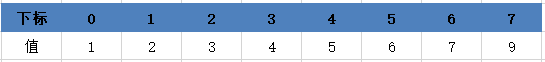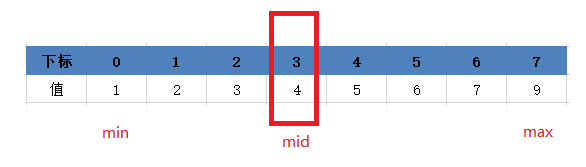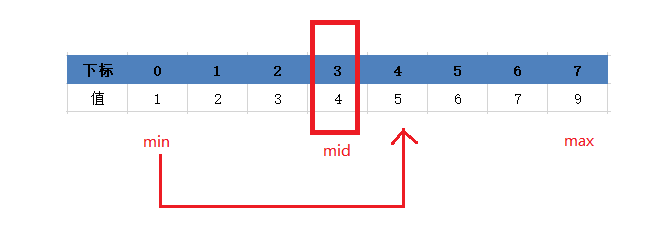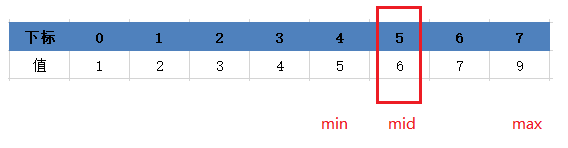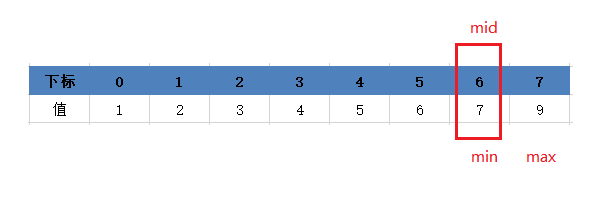import java.util.Arrays;
public class HalfSearch{

public static void main(String[] args){
int array[] = {1,4,2,3,6,5,7,9};
Arrays.sort(array);
int num = 7;
int min=0;
int mid=0;
int max=array.length-1;
while(min<=max){
mid=(min+max)/2;
if(num<array[mid]){
max=mid-1;
}else if(num>array[mid]){
min=mid+1;
}else {
System.out.print("找到这个数"+num);
return;
}
}System.out.print("找不到这个数"+num);
}


©️2019 CSDN 皮肤主题: 1024 设计师: 上身试试